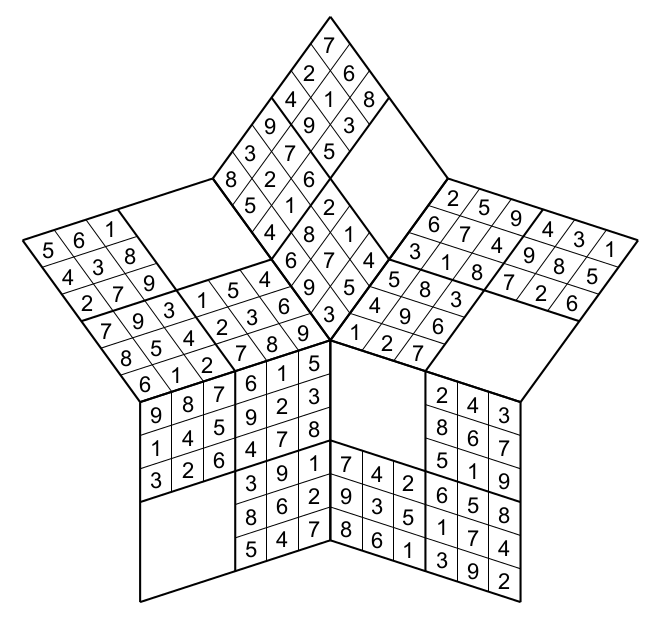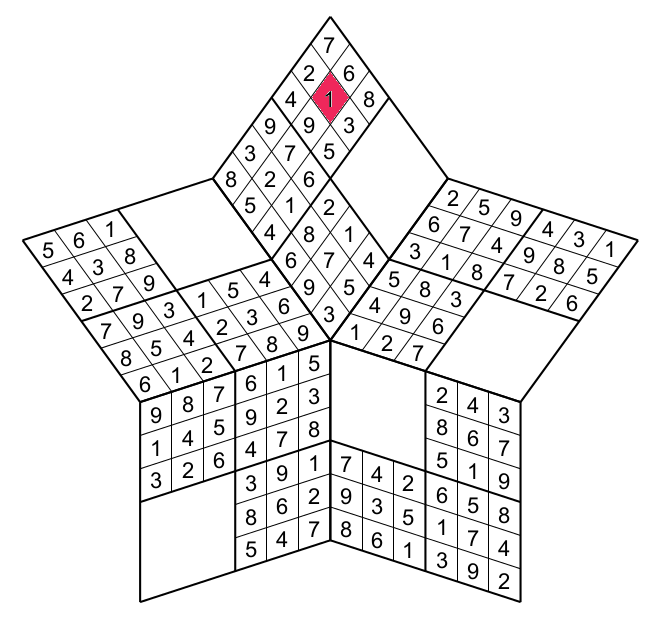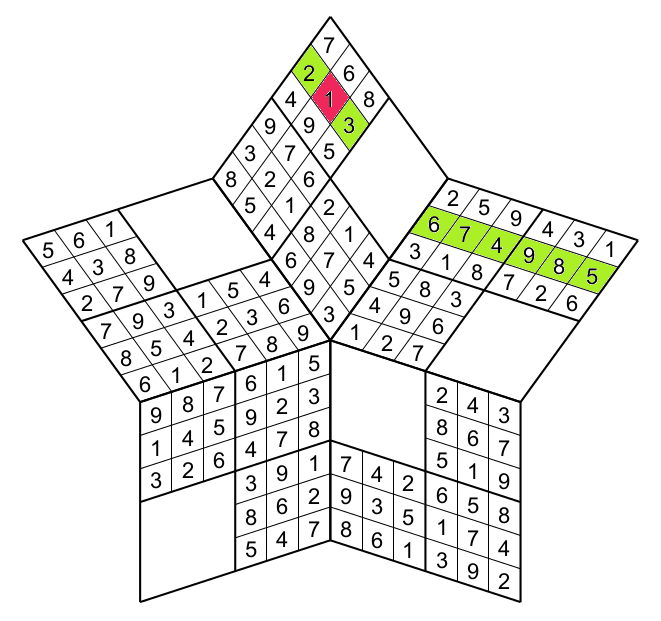Sparkube's Rules

## Sparkube's Rules

The Sparkube puzzle is a cross between the SparkSudoku puzzle (Rules of Spark Sudoku) and the Sudokube puzzle (SudoKube). This puzzles could take four different shapes : pentagram (star with 5 points), hexagram (6), heptagram(7) and octagram(8) . This is a pentagram## RULES

The rules are the same than the standard Sudoku: fill each cell with number from 1 to 9 such that each row,column and region has each number.Instead of playing on a square grid you play on a star. A row or a column begins in on one point of the star and finishes on the adjacent Each column (or rwo) contains 3 (full) regions). Each point of the star has four regions: three full and one blank. You have to ihnore the empty region when you follow a row/column. By examplefigure 1

The cell having the number 3 (red), pertains to the green row,figure 2

the green column,figure 3

and of course to the green region.figure 4

Finaly the region, row and column that the red cell belongs.figure 5

This is the three other shapes:

You will be thrilled by this new puzzle, the best way to know it is to try it !!